# Rigid body game physics 6

A realistic simulation requires the estimation of friction forces.

## Friction Forces

Colliding as well as resting contacts cause constraint impulses as well as friction impulses. So far we have only estimated the constraint impulses of colliding and resting contacts. Constraint impulses are in the direction of the contact's normal vector. Friction impulses act tangential to the contact plane (orthogonal to the normal vector). The inequality constraint isJ and b have two rows. The vectors t1 and t2 are chosen so that they are orthogonal to the normal vector n and orthogonal to each other. Friction forces try to reduce tangential velocities to zero. I.e. b isThe linear components of J are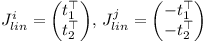and the angular components of J are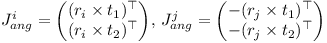As before λ is computed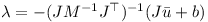Here λ has two elements. λ1 and λ2 are scaled with the same positive factor if necessary so that the length of the 2D vector consisting of λ1 and λ2 is smaller than the normal impulse Pn multiplied with the friction constant μ.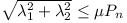Basically the overall force vector needs to reside in the friction cone. In a similar fashion as for constraint impulses, friction impulses are subtracted, recomputed, and then added to the accumulated impulses P of the two objects, when iterating.If you have made it this far, you know how to build a small physics engine!

Any feedback, comments, and suggestions are welcome.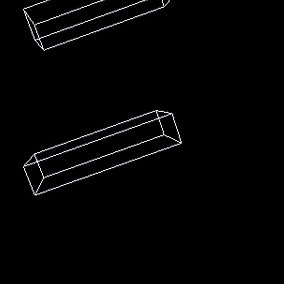# Rigid body game physics 5

In order to handle collisions and resting contacts, it is necessary to determine contact points. One can achieve this using the separating plane algorithm (also see Baraff).

## Separating Planes Algorithm

The separating plane algorithm only applies to convex polyhedra. However non-convex objects can in many cases be approximated by a union of convex polyhedra. A separating plane is a plane such that all points of the polyhedra A and B lie on opposite sides of the plane. If two polyhedra are not intersecting, a separating plane can either be defined using one of the faces of one of the polyhedra or be constructed from one edge of A and one edge of B.

The distance d of a point x from the plane P(p,n) defined by the point p and the normal vector n is: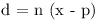1. In the case of using a face from polyhedron A, the point p is a corner of the face. The normal vector n is the normalized cross product of two edges of the face and pointing outward from the polyhedron. The point x of polyhedron B with the smallest distance d defines the distance of B to the face of A. If this distance is positive, the face of polyhedron A is a separating plane.

2. In the case of using two edges, the point p is the corner of an edge from polyhedron A. The normal vector n is the positive or negative normalized cross product of the two edge vectors. Again the point x of polyhedron B with the smallest distance d defines the distance of B to the face of A.

From all the planes, the one with the largest distance d is selected. If the distance is zero or negative, the two polyhedra are in contact. One can place a plane in the "middle" of the contact by moving it by half the distance d, i.e.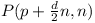In the next step all points from A and B, which have a distance less than ε to the plane, are selected. Two vectors u and v orthogonal to the normal n and orthogonal to each other are determined. The points are projected onto the plane and represented using the vectors u and v: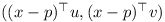The convex hull of the each of the two polygons is determined. Then the intersection of the resulting two 2D polygons is computed. Finally the convex hull of the intersection is taken. The points are projected back into 3D space. These are the contact points used for simulating the colliding and resting contacts.

# Rigid body game physics 4

The following article is partially based on Hubert Eichner's article on inequality constraints.

## Inequality Constraints for Colliding Contacts

At a colliding contact the relative speed of the two objects is negative. I.e. a contact is a colliding (and not a resting) contact if the normal speed at the contact point is below a small negative threshold. Colliding contacts have restitution, i.e. the collision is partially elastic and the two objects will separate after the collision. The colliding contacts and affected joints are handled using a special time step of zero duration. Again the inequality constraint isThe linear and angular components of J are the same as for a resting contact. The correction term b however depends on the initial normal speed at the contact point vn and the restitution coefficient ε: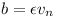## Sequential Impulses for Collisions

Again the contact and joint impulses are estimated iteratively. Note that the normal speed vn at each contact is determined beforehand and stays constant during the iterations.

• determine vn for all colliding contacts
• for each iteration
• for each joint
1. compute Jacobian J and correction vector b
2. predict speed u
3. compute λ
4. compute the impulse P
5. add P to accumulated impulses of the two objects
• for each colliding contact
1. subtract old impulses P from previous iteration from accumulated impulses of the two objects
2. compute Jacobian J and correction vector b
3. predict speed u
4. compute new λ and clip it to be greater or equal to zero
5. compute the impulse P
6. add P to accumulated impulses of the two objects
• apply impulses to objects

The value λ is stored as Pn for limiting friction impulses lateron. The time step here is zero! Therefore external forces do not need to be considered while handling collisions. An object falling to the floor will experience several collisions until the linear and angular speed has decreased sufficiently for the contacts to become resting contacts.# Rigid body game physics 3

The following article is based on Hubert Eichner's article on inequality constraints.

## Inequality Constraints for Resting Contacts

Contact points (resting contacts) are represented as inequality constraints. In contrast to a joint, a resting contact can only create repellent forces and no attracting forces. Similar to a joint constraint, the resting contact is represented using a function C(y(t)). Here C is one-dimensional and it is basically the distance of the two objects at the contact point. The inequality constraint of the resting contact iswith the matrix J having one row and twelve columns. Instead of anchor points, one uses vectors ri and rj from the center of each object to the contact point. Using the contact normal n the inequality constraint becomes: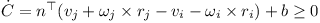The rotational component can be simplified as shown below: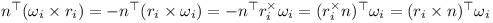Thus the linear components of J are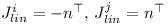And the angular components of J areThe correction term depends on the distance d. I.e. if the distance is negative, a correction is required so that the objects do not penetrate each other any more.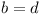## Sequential Impulses (updated)

The impulses generated by contact constraints and joint constraints are accumulated in a loop. The (cumulative) λ obtained for contact constraint furthermore is clipped to be greater or equal to zero. Note that it has to be the cumulative value which is clipped. The algorithm for solving the joint and contact constraints becomes:

• for each iteration
• for each joint
1. compute Jacobian J and correction vector b
2. predict speed u
3. compute λ
4. compute the impulse P
5. add P to accumulated impulses of the two objects
• for each resting contact
1. subtract old impulses P from previous iteration from accumulated impulses of the two objects
2. compute Jacobian J and correction vector b
3. predict speed u
4. compute new λ and clip it to be greater or equal to zero
5. compute the impulse P
6. add P to accumulated impulses of the two objects
• use impulses and external forces to perform Runge Kutta integration step

The sequential impulse iterations have to be performed four times when using the Runge-Kutta method. The value λ is stored as Pn for limiting friction impulses lateron.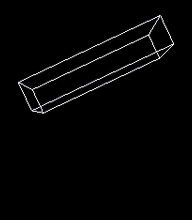# Rigid body game physics 2

The following article is heavily based on Hubert Eichner's article on equality constraints. The math for the joint types was taken from Kenny Erleben's PhD thesis.

## Constraint Impulses

A constraint between two objects is specified as a multi-dimensional function C(y(t)). The constrained is fulfilled by attempting to keep each component of the derivative of C at zero:J is the Jacobian matrix and u is a twelve-element vector containing the linear and rotational speed of the two bodies. Here the derivative of the orientation is represented using the rotational speed ω.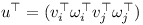The two objects without a connecting joint would together have twelve degrees of freedom. Let's assume that the two objects are connected by a hinge joint. In this case the function C has five dimensions because a hinge only leaves seven degrees of freedom. The Jacobian J has five rows and twelve columns. In practise an additional vector b is used to correct for numerical errors.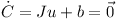The twelve-element constraint impulse P does not do any work and therefore must be orthogonal to u.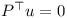The rows of J span the orthogonal space to u. Therefore P can be expressed as a linear combination of the rows of J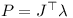where λ is a vector with for example five elements in the case of the hinge joint.

Given an initial estimate for u and the constraint impulse P one can compute the final velocity u.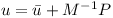M is the twelve by twelve generalised mass matrix which contains the mass and inertia tensors of the two objects.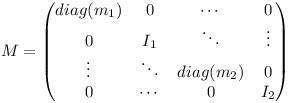Inserting the equation for u into the constraint equation yields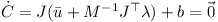This equation can be used to determine λλ then can be used to determine the constraint impulse P! When performing the numerical integration, the external forces (multiplied with the timestep) and the constraint impulse are used to determine the change in linear and rotational speed of the two bodies.

## Joints

### Ball-in-Socket Joint

The matrix J for bodies i and j and the velocity vector u can be split up into the linear and angular parts.v is the three-dimensional linear speed and ω is the rotational speed of the individual object. At the anchor point of the joint the speed of the two rigid bodies must be the same:Thus the linear components of J are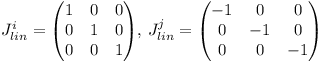and the angular components of J are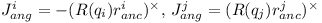where the cross-product matrix of a vector is defined as follows: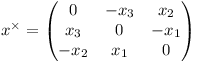The correcting vector b simply corrects for the offset at the anchor point.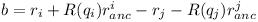### Hinge Joint

A hinge joint has the constraints of the ball-in-socket joint and two additional angular constraints. I.e. there are five constraints altogether.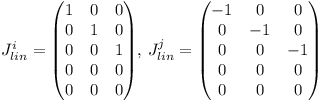The rotation axis in world coordinates can be computed from the average of the two rotated axes which should be coinciding under ideal circumstances.The relative rotation of the two rigid bodies must be parallel to the axis s of the hinge. Given two vectors t1 and t2 orthogonal to the rotation axis of the hinge joint, the two additional constraints are: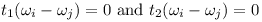Therefore the angular parts of the Jacobian are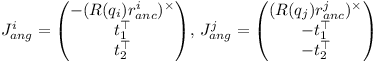The error u of axis misalignment is the cross product of the two rotated axes.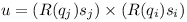The error u is orthogonal to the rotation axis s. It is projected onto the vectors t1 and t2 when computing the correction vector b: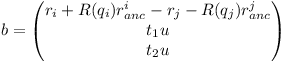See Kenny Erleben's PhD thesis for these and other types of joints (slider joint, hinge-2 joint, universal joint, fixed joint).

## Sequential Impulses

To fulfill multiple constraints, an algorithm called sequential impulses is used. Basically the constraint impulses are updated a few times until the impulses become stable.

• for each iteration
• for each joint
1. compute Jacobian J and correction vector b
2. predict speed u
3. compute λ
4. compute the impulse P
5. add P to accumulated impulses of the two objects
• use impulses and external forces to perform Runge Kutta integration step

The sequential impulse iterations have to be performed four times when using the Runge-Kutta method.